—— 青面对隼飞暗夜密语》，第156页

Barkface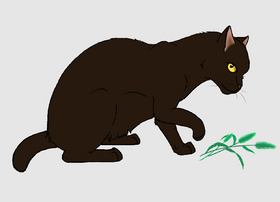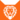狮族 (Q646)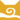风族 巫医风族 首席巫医
（继任自鹰心 (Q3430)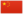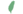小树皮、小青 Barkkit  小吠
 树皮爪、青爪 Barkpaw  吠掌
 树皮脸、青面 Barkface  吠臉

Q3008：风族公猫，自《预言开始》系列登场的角色

## 细节

### 外貌

• 他是一只黄色眼睛、口鼻泛灰、:18尾巴很短:猫物表的暗棕色公猫。:8

### 作者声明

• Su[?]认为他是因为年纪而自然死亡。

### 趣闻

• 他陪同高星进行九命仪式，:45章但当时他的导师、作为首席巫医的鹰心仍然建在。:1章

### 勘误

• 他和小鼩鼱曾被描述为“比小高年长三个月”，:1章但之后又提到他“比小高年长两个月”。:3章
• 在《黄牙的秘密》中，青爪和奔爪一起受訓，但他比奔鼻的导师黄牙还要年長。:366

## 语录

—— 高星对青面预言的反应，《寒冰烈火》正体中文版，第80页

—— 风族祖灵警告隼飞，《暗夜密语》，第155-157页

## 登场书目

• 寒冰烈火 (Q59)[配角][首次登场🐱]
• 疑云重重 (Q60)[猫物闲角]
• 风起云涌 (Q65)[猫物闲角]
• 险路惊魂 (Q66)[配角]
• 力挽狂澜 (Q67)[配角]
• 午夜追踪 (Q139)[配角]
• 新月危机 (Q140)[配角]
• 重现家园 (Q141)[配角]
• 星光指路 (Q142)[配角]
• 黄昏战争 (Q143)[配角]
• 日落和平 (Q144)[配角]
• 预视力量 (Q152)[配角]
• 暗河汹涌 (Q153)[配角]
• 驱逐之战 (Q154)[猫物闲角]
• 天蚀遮月 (Q155)[配角]
• 暗夜长影 (Q156)[配角]
• 拂晓之光 (Q157)[配角]
• 第四学徒 (Q158)[对话提及][死亡时刻✝]
• 暗夜密语 (Q160)[配角]
• 月光印记 (Q161)[配角]
• 学徒探索 (Q170)[配角]
• 火星的探索 (Q176)[猫物闲角]
• 黄牙的秘密 (Q180)[配角]
• 高星的复仇 (Q181)[配角]
• 鸦羽的拷问 (Q186)[对话提及]
• 灰条的誓言 (Q4057)[猫物闲角]
• 豹星的荣耀 (Q4426)[猫物闲角]
• 冬青叶的故事 (Q187)[猫物闲角]
• 虎掌的愤怒 (Q190)[猫物闲角]
• 叶池的祈愿 (Q191)[猫物闲角]
• 红尾的恩债 (Q3565)[配角]
• 蛾翅的秘密 (Q3628)[配角]
• 黑脚的清算 (Q4336)[仅于回忆]
• 族群决定 (Q4580)[配角]
• ## 外部链接

### 官方文章

• 解读征兆 （提及）
• Mudclaw's Rebellion （提及）
• ## 脚注

1. [待补充]
2. 同上。
引用错误：在`<references>`中以“otherName”名字定义的`<ref>`标签没有在先前的文字中使用。

## 参考文献

这篇文章基于CC BY-SA 3.0许可使用了猫武士维基（英语）Barkface一文中的部分内容。
0.0
0人评价